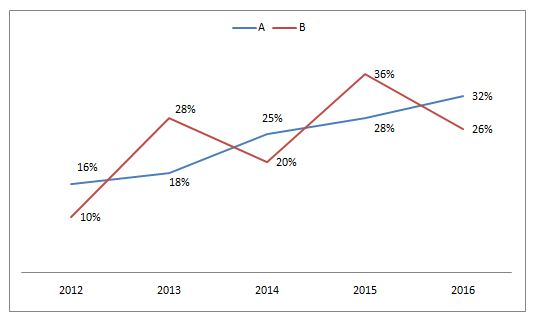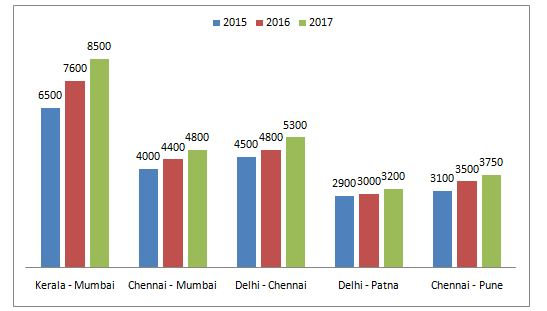# Quantitative Aptitude Questions (Data Interpretation) for SBI Clerk/PO Prelims 2018 Day-136

Dear Readers, SBI is conducting Online Examination for the recruitment of Clerical Cadre and probationary officer. To enrich your preparation here we have providing new series of Data Interpretation – Quantitative Aptitude Questions. Candidates those who are appearing in SBI Clerk/PO Prelims Exams can practice these Quantitative Aptitude average questions daily and make your preparation effective.

[WpProQuiz 2409]

Click “Start Quiz” to attend these Questions and view Solutions

Directions (Q. 1 – 5) Study the following information carefully and answer the given questions:

Following line graph shows the % profit of two companies A and B in different years.

Profit % = [(Income – Expenditure)/Expenditure]*1001. If the expenditure of Company A in the year 2012 is 32 lakhs and the income of Company B in the year 2014 is 13 lakhs more than the expenditure of company A in the year 2012, then the income of company A in 2012 is approximately what percentage of the expenditure of company B in the year 2014?
1. 112 %
2. 94 %
3. 100 %
4. 88 %
5. 124 %
1. If the expenditure of company A in the year 2013 and the expenditure of company B in the year 2015 is same and the income of company A in the year 2013 is 44.25 lakhs, then find the income of company B in the year 2015?
1. 63 lakhs
2. 51 lakhs
3. 47 lakhs
4. 39 lakhs
5. None of these
1. If the income of company A and B in the year 2014 is same, then the ratio of expenditure of company A and B in the year 2014 is?
1. 24 : 25
2. 16 : 23
3. 31 : 39
4. 8 : 11
5. None of these
1. If the income of company B in the year 2016 is 37.8 lakhs and the expenditure of company A in the year 2015 is 27 lakhs, then the expenditure of company B in the year 2016 is approximately what percentage more/less than the income of company A in the year 2015?
1. 24 % more
2. 32 % more
3. 8 % less
4. 13 % less
5. 18 % more
1. If the expenditure of company A and B in the year 2015 is 27 lakhs and 33 lakhs respectively, then find the difference between the income of company A and B in the year 2015?
1. 11.44 lakhs
2. 15.78 lakhs
3. 10.32 lakhs
4. 18.15 lakhs
5. None of these

Directions (Q. 6 – 10) Study the following information carefully and answer the given questions:

The bar graph shows the airfare (in Rs.) for various routes in different years.1. Find the difference between the total airfare in 2015 to that of total airfare in 2017 for all the given routes together?
1. Rs. 4550
2. Rs. 5680
3. Rs. 6420
4. Rs. 3490
5. None of these
1. Find the ratio between the total airfare of Delhi – Chennai route to that of Chennai – Mumbai for all the given years together?
1. 56 : 75
2. 45 : 77
3. 73 : 66
4. 65 : 41
5. None of these
1. Total airfare in the year 2016 for the routes of Chennai – Mumbai, Delhi – Patna and Chennai – Pune together is approximately what percentage of total airfare in the year 2017 for the routes of Kerala – Mumbai, Delhi – Chennai and Chennai – Pune together?
1. 50 %
2. 62 %
3. 77 %
4. 86 %
5. 45 %
1. Find the average airfare of all the given routes together in the year 2016?
1. 5170
2. 6340
3. 3890
4. 4660
5. None of these
1. Total airfare in the year 2017 is approximately what percentage more than the total airfare in the year 2016, for all the given routes together?
1. 18 %
2. 10 %
3. 27 %
4. 33 %
5. 45 %

The expenditure of Company A in the year 2012 = 32 lakhs

The income of Company B in the year 2014 = 13 + 32 = 45 lakhs

The income of company A in 2012

= > 16 = [(I – 32)/32]*100

= > 512 + 3200 = 100I

= > I = 3712/100 = 37.12 lakhs

The expenditure of Company B in the year 2014

= > 20 = [45 – e)/e]*100

= > 20e = 4500 – 100e

= > 120e = 4500

= > e = (4500/120) = 37.5 lakhs

Required % = (37.12/37.5)*100 = 98.98 % = 100 %

The income of company A in the year 2013 = 44.25 lakhs

The expenditure of company A in the year 2013

= > 18 = [(44.25 – e)/e]*100

= > 118e = 4425

= > e = (4425/118) = 37.5 lakhs

The expenditure of company A in the year 2013 = The expenditure of company B in the year 2015

The expenditure of company B in the year 2015 = 37.5 lakhs

The income of company B in the year 2015

= > 36 = [(I – 37.5)/37.5]*100

= > 1350 = 100I – 3750

5100 = 100I

I = 51 lakhs

Let the income of company A and B in the year 2014 be x,

The expenditure of company A in the year 2014

= > 25 = [(x – E1)/E1]*100

= > 125E1 = 100x

= > E1 = (100x/125) = (4x/5)

The expenditure of company B in the year 2014

= > 20 = [(I – E2)/E2]*100

= > 120E2 = 100x

= > E2 = (100x/120) = (5x/6)

Required ratio

= > (4x/5): (5x/6)

= > 24: 25

The income of company B in the year 2016 = 37.8 lakhs

The expenditure of company B in the year 2016

= > 26 = [(37.8 – e)/e]*100

= > 126e = 3780

= > e = (3780/126) = 30 lakhs

The expenditure of company A in the year 2015 = 27 lakhs

The income of company A in the year 2015

= > 28 = [(I – 27)/27]*100

= > 756 = 100I – 2700

= > 3456 = 100I

= > I = 34.56 lakhs

Required % = [(34.56- 30)/34.56]*100 = 13.19 % = 13 % less

The expenditure of company A in the year 2015 = 27 lakhs

The income of company A in the year 2015

= > 28 = [(I – 27)/27]*100

= > 756 = 100I – 2700

= > 3456 = 100I

= > I = 34.56 lakhs

The expenditure of company B in the year 2015 = 33 lakhs

The income of company B in the year 2015

= > 36 = [(I – 33)/33]*100

= > 1188 = 100I – 3300

= > I = (4488/100) = 44.88 lakhs

Required difference = 44.88 – 34.56 = 10.32 lakhs

Direction (6-10)

The total airfare in 2015 for all the given routes together

= > 6500 + 4000 + 4500 + 2900 + 3100

= > 21000

The total airfare in 2017 for all the given routes together

= > 8500 + 4800 + 5300 + 3200 + 3750

= > 25550

Required difference = 25550 – 21000 = Rs. 4550

The total airfare of Delhi – Chennai route for all the given years together

= > 4500 + 4800 + 5300 = 14600

The total airfare of Chennai – Mumbai for all the given years together

= > 4000 + 4400 + 4800 = 13200

Required ratio = 14600: 13200 = 73: 66

Total airfare in the year 2016 for the routes of Chennai – Mumbai, Delhi – Patna and Chennai – Pune together

= > 4400 + 3000 + 3500 = 10900

Total airfare in the year 2017 for the routes of Kerala – Mumbai, Delhi – Chennai and Chennai – Pune together

= > 8500 + 5300 + 3750 = 17550

Required % = (10900/17550)*100 = 62.108 % = 62 %

The total airfare of all the given routes together in the year 2016

= > 7600 + 4400 + 4800 + 3000 + 3500

= > 23300

Required average = (23300/5) = 4660

Total airfare in the year 2017 for all the given routes together

= > 8500 + 4800 + 5300 + 3200 + 3750

= > 25550

Total airfare in the year 2016 for all the given routes together

= > 7600 + 4400 + 4800 + 3000 + 3500

= > 23300

Required % = [(25550 – 23300)/23300]*100 = 9.656 % = 10 %

Daily Practice Test Schedule | Good Luck

 Topic Daily Publishing Time Daily News Papers & Editorials 8.00 AM Current Affairs Quiz 9.00 AM Quantitative Aptitude “20-20” 11.00 AM Vocabulary (Based on The Hindu) 12.00 PM General Awareness “20-20” 1.00 PM English Language “20-20” 2.00 PM Reasoning Puzzles & Seating 4.00 PM Daily Current Affairs Updates 5.00 PM Data Interpretation / Application Sums (Topic Wise) 6.00 PM Reasoning Ability “20-20” 7.00 PM English Language (New Pattern Questions) 8.00 PM